Math Concepts
Geometry Theorems
96k views

 1 Introduction 2 Geometry Theorems 3 Angle Theorems 4 Triangle Theorems 5 Circle Theorems 6 Parallelogram Theorems 7 Summary 8 FAQs

21 October  2020

## Introduction

Geometry is a very organized and logical subject. The guiding light for solving Geometric problems is Definitions, Geometry Postulates, and Geometry Theorems. So before moving onto the geometry theorems list, let us discuss these to aid in geometry postulates and theorems list.

Definitions are what we use for explaining things.

E.g.: - You know that a circle is a round figure but did you know that a circle is defined as lines whose points are all equidistant from one point at the center.

Or did you know that an angle is framed by two non-parallel rays that meet at a point?

This is what is called an explanation of Geometry.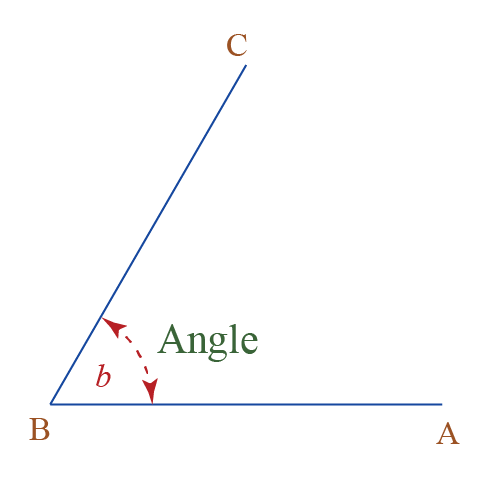### Postulates

Geometry Postulates are something that can not be argued. It’s like set in stone.
Example: - For 2 points only 1 line may exist. It is the postulate as it the only way it can happen. Or when 2 lines intersect a point is formed.We can also say Postulate is a common-sense answer to a simple question.

### Theorems

Unlike Postulates, Geometry Theorems must be proven. To prove a Geometry Theorem we may use Definitions, Postulates, and even other Geometry theorems.

For example: If I say two lines intersect to form a 90° angle, then all four angles in the intersection are 90° each.

## Geometry Theorems

Key components in Geometry theorems are Point, Line, Ray, and Line Segment. Let us go through all of them to fully understand the geometry theorems list.

### Point

In maths, the smallest figure which can be drawn having no area is called a point.

### Line

A straight figure that can be extended infinitely in both the directions

### Ray

A line having one endpoint but can be extended infinitely in other directions.

### Line Segment

A line having two endpoints is called a line segment.

Now let’s discuss the Pair of lines and what figures can we get in different conditions.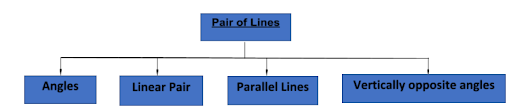### 1. Angles

Two rays emerging from a single point makes an angle.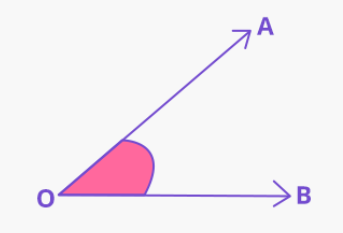### 2. Linear Pair

When two or more than two rays emerge from a single point. Then the angles made by such rays are called linear pairs.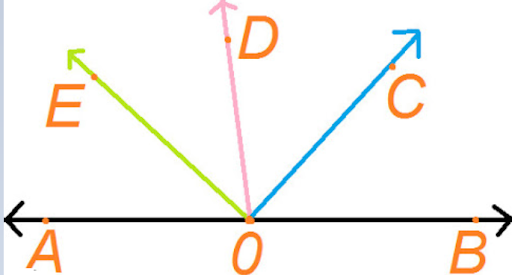### 3. Parallel Lines

When the perpendicular distance between the two lines is the same then we say the lines are parallel to each other.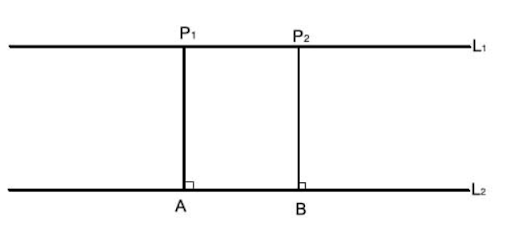### 4. Vertically opposite angles

If there are two lines crossing from one particular point then the opposite angles made in such a condition are equals.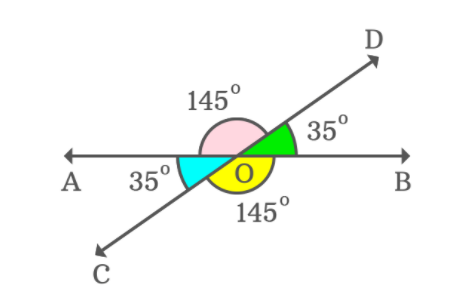Now that we are familiar with these basic terms, we can move onto the various geometry theorems. To make it easier to connect and hence apply, we have categorized them according to the shape the geometry theorems apply to.

## Angle Theorems

The relation between the angles that are formed by two lines is illustrated by the geometry theorems called “Angle theorems”. Some of the important angle theorems involved in angles are as follows:

### 1. Alternate Exterior Angles Theorem

When two parallel lines are cut by a transversal then resulting alternate exterior angles are congruent.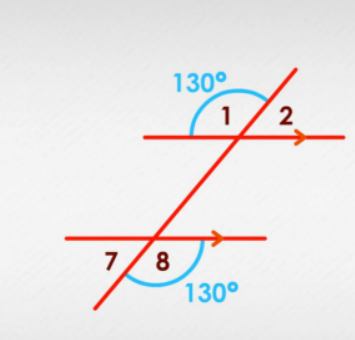The alternate exterior angles have the same degree measures because the lines are parallel to each other.

### 2. Alternate Interior Angles Theorem

When two parallel lines are cut by a transversal then resulting alternate interior angles are congruent.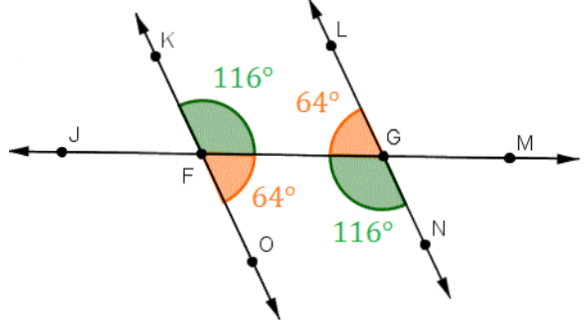The alternate interior angles have the same degree measures because the lines are parallel to each other.

One way to find the alternate interior angles is to draw a zig-zag line on the diagram.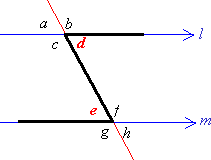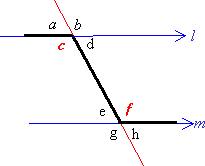### 3. Congruent Complements Theorem

If two angles are complementary to the same angle or of congruent angles, then the two angles are congruent.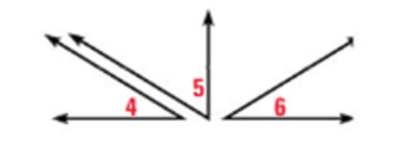### 4. Congruent Supplements Theorem

If two angles are supplements to the same angle or of congruent angles, then the two angles are congruent.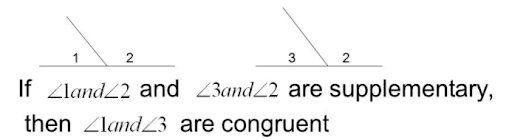### 5.  Right Angles Theorem

If two angles are both supplement and congruent then they are right angles.### 6.  Same-Side Interior Angles Theorem

If two parallel lines are cut by a transversal, then the interior angles on the same side of the transversal are supplementary.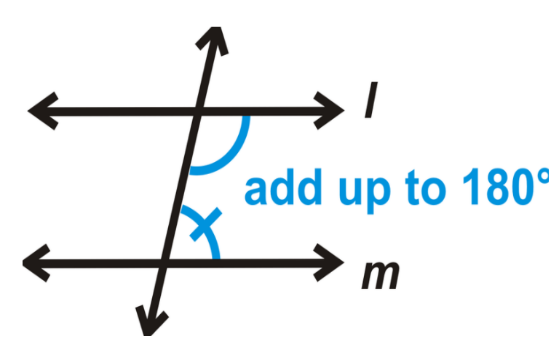### 7.  Vertical Angles Theorem

Angles that are opposite to each other and are formed by two intersecting lines are congruent.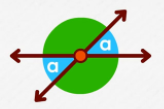Now let us move onto geometry theorems which apply on triangles.

## Triangle Theorems

We know that there are different types of triangles based on the length of the sides like a scalene triangle, isosceles triangle, equilateral triangle and we also have triangles based on the degree of the angles like the acute angle triangle, right-angled triangle, obtuse angle triangle.

Though there are many Geometry Theorems on Triangles but Let us see some basic geometry theorems.

### Theorem 1

In any triangle, the sum of the three interior angles is 180°.

 Example

Suppose XYZ are three sides of a Triangle, then as per this theorem; ∠X + ∠Y + ∠Z = 180°

### Theorem 2

If a side of the triangle is produced, the exterior angle so formed is equal to the sum of corresponding interior opposite angles.

 Example

For a triangle, XYZ, ∠1, ∠2, and ∠3 are interior angles. And ∠4, ∠5, and ∠6 are the three exterior angles.

### Theorem 3

The base angles of an isosceles triangle are congruent.

Suppose a triangle XYZ is an isosceles triangle, such that;

XY = XZ [Two sides of the triangle are equal]

Hence,

∠Y = ∠Z

Where ∠Y and ∠Z are the base angles.

Now Let’s learn some advanced level Triangle Theorems.

Theorem 3: If a line is drawn parallel to one side of a triangle to intersect the midpoints of the other two sides, then the two sides are divided in the same ratio.

 Example

XYZ is a triangle and L M is a line parallel to Y Z such that it intersects XY at l and XZ at M.

Hence, as per the theorem:

XL/LY = X M/M Z

### Theorem 4

If a line divides any two sides of a triangle in the same ratio, then the line is parallel to the third side.

Suppose XYZ is a triangle and a line L M divides the two sides of triangle XY and XZ in the same ratio, such that;

XL/LY = X M/M Z

### Theorem 5

If in two triangles, corresponding angles are equal, then their corresponding sides are in the same ratio and hence the two triangles are similar.

Let ∆ABC and ∆PQR are two triangles.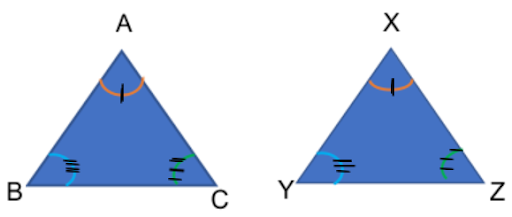Then as per the theorem,

AB/PQ = BC/QR = AC/PR (If ∠A = ∠P, ∠B = ∠Q and ∠C = ∠R)

And ∆ABC ~ ∆PQR

### Theorem 6

If in two triangles, the sides of one triangle are proportional to other sides of the triangle, then their corresponding angles are equal and hence the two triangles are similar.

Let us now proceed to discussing geometry theorems dealing with circles or circle theorems.

## Circle Theorems

Circle theorems helps to prove the relation of different elements of the circle like tangents, angles, chord, radius, and sectors. Or we can say circles have a number of different angle properties, these are described as circle theorems.

Now let’s study different geometry theorems of the circle.

### Circle Theorems 1

Angles in the same segment and on the same chord are always equal.### Circle Theorems 2

A line drawn from the center of a circle to the mid-point of a chord is perpendicular to the chord at 90°.### Circle Theorems 3

The angle at the center of a circle is twice the angle at the circumference.### Circle Theorems 4

The angle between the tangent and the side of the triangle is equal to the interior opposite angle.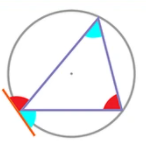### Circle Theorems 5

The angle in a semi-circle is always 90°.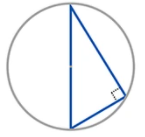### Circle Theorems 6

Tangents from a common point (A) to a circle are always equal in length. AB=BC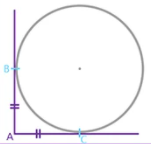### Circle Theorems 7

The angle between the tangent and the radius is always 90°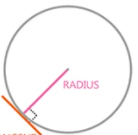### Circle Theorems 8

In a cyclic quadrilateral, all vertices lie on the circumference of the circle. Opposites angles add up to 180°.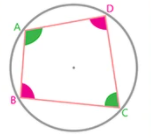Proceed to the discussion on geometry theorems dealing with paralellograms or parallelogram theorems.

## Parallelogram Theorems

A parallelogram is a quadrilateral with both pairs of opposite sides parallel.

Let’s now understand some of the parallelogram theorems.

### Parallelogram Theorems 1

If both pairs of opposite sides of a quadrilateral are congruent, then the quadrilateral is a parallelogram.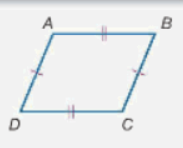### Parallelogram Theorems 2

If both pairs of opposite angles of a quadrilateral are congruent, then the quadrilateral is a parallelogram.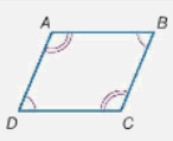### Parallelogram Theorems 3

If the diagonals of a quadrilateral bisect each other, then the quadrilateral is a parallelogram.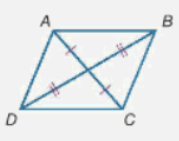### Parallelogram Theorems 4

If one pair of opposite sides of a quadrilateral is both parallel and congruent, then the quadrilateral is a parallelogram.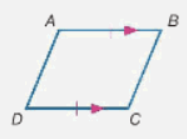## Summary

In Geometry, you learn many theorems which are concerned with points, lines, triangles, circles, parallelograms, and other figures. Geometry Theorems are important because they introduce new proof techniques.

You must have heard your teacher saying that Geometry Theorems are very important but have you ever wondered why? We leave you with this thought here to find out more until you read more on proofs explaining these theorems. Proving the geometry theorems list including all the angle theorems, triangle theorems, circle theorems and parallelogram theorems can be done with the help of proper figures.

Written by Rashi Murarka

## Which of the following states the pythagorean theorem?

The Pythagorean theorem consists of a formula a^2+b^2=c^2 which is used to figure out the value of (mostly) the hypotenuse in a right triangle. The a and b are the 2 "non-hypotenuse" sides of the triangle (Opposite and Adjacent).

## What is the vertical angles theorem?

Angles that are opposite to each other and are formed by two intersecting lines are congruent.

Related Articles